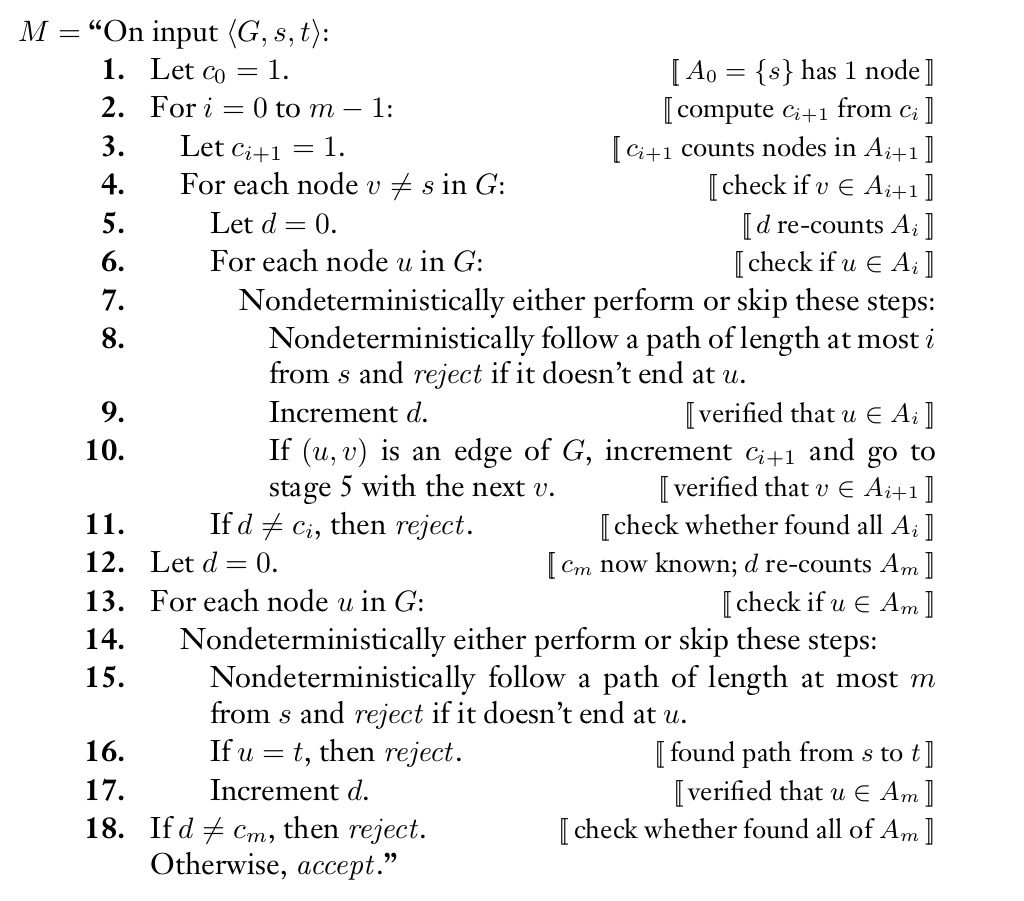## [ITOC-ch8] SPACE COMPLEXITY

Space somplexity

• M is deterministic
• f(n) is the maximum number of tape cells that M scans on any input of length n.

nondeterministic Space complexity

• M is nondeterministic
• f(n) is the maximum number of tape cells that M scans on any branch on any input of length n.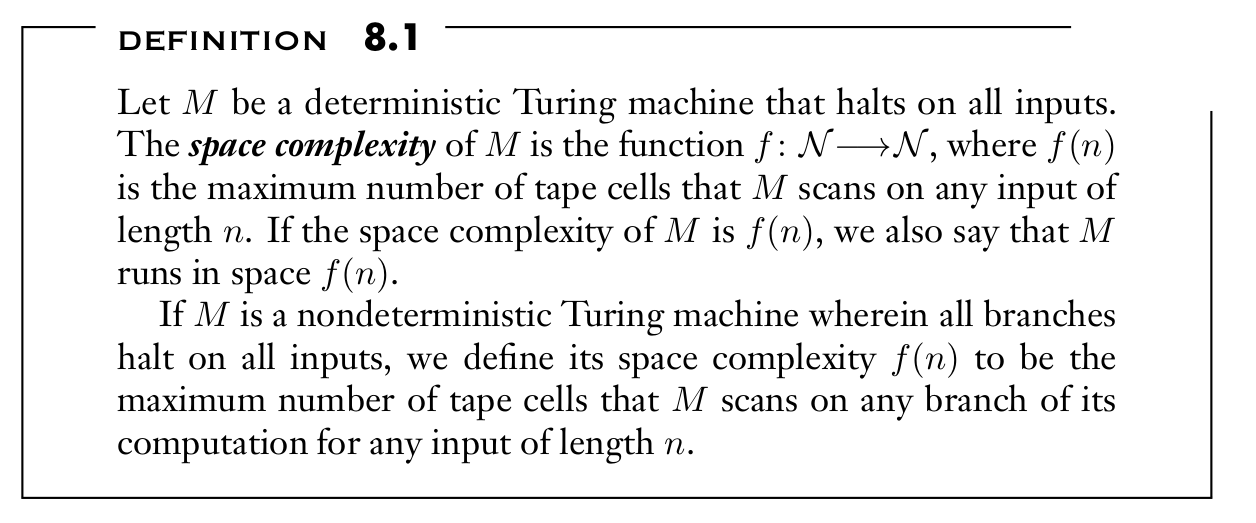SPACE(f(n))

• collection of languages that are decidable by a TM

NSPACE(f(n))

• collection of languages that are decidable by a NTM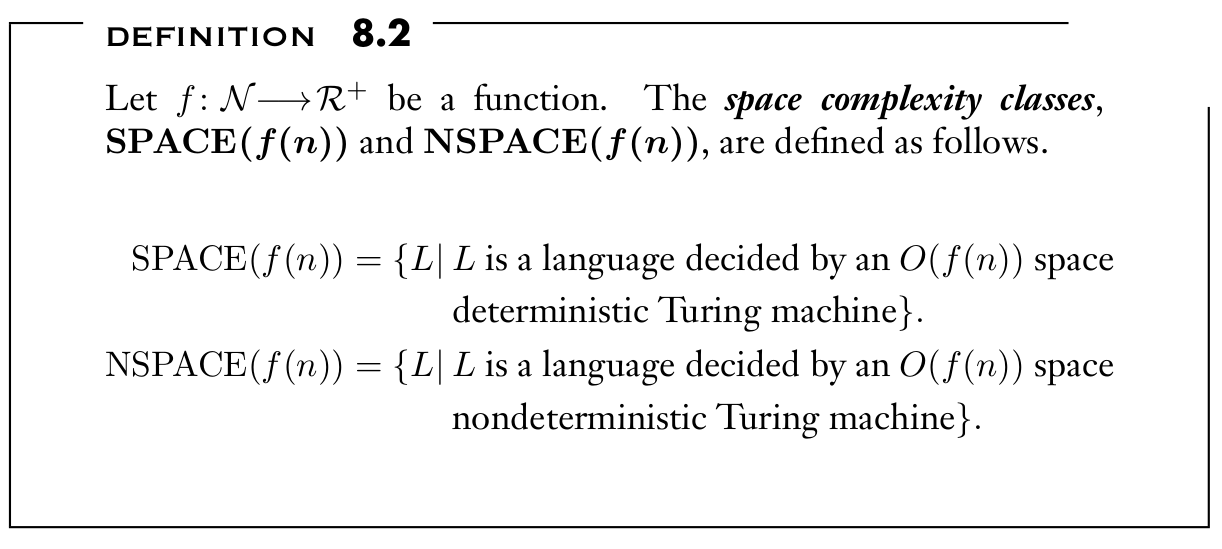Examples

### 8.1 SAVITCH’S THEOREM

NTM uses $f(n)$ space $==$ TM uses $f^2(n)$ space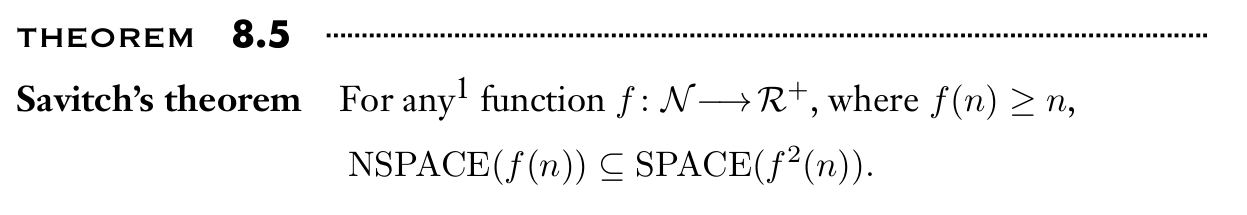Proof

• yieldability problem: NTM can get from $c1$ to $c2$ within t steps using only f (n) space.
• recursive: space for storing the recursive stack is $logt = log ( 2 ^ {(Of(n))}) = O(f(n))$
• Each level of the recursion uses $O(f (n))$ space to store a configuration.
• Hence the deterministic simulation uses $O(f^2(n))$ space.
•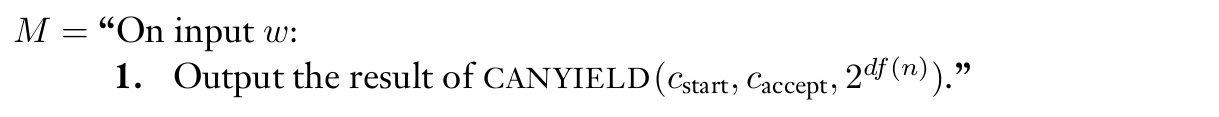•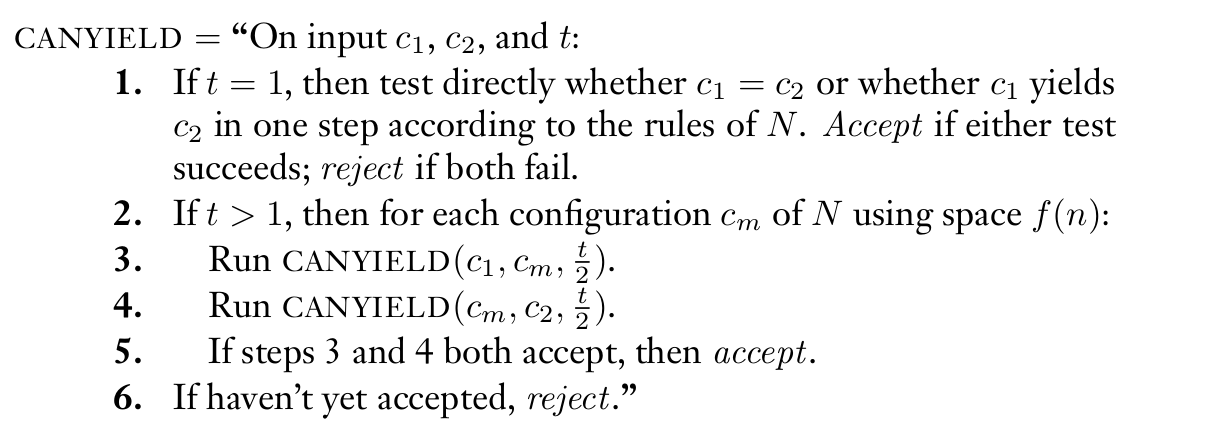• technical difficulty to know $f(n)$: tries $f(n) = 1,2,3…$
• accept when accept configuration is reachable
• reject when no configuration of length $i + 1$ is reachable

### 8.2 THE CLASS PSPACE

class PSPACE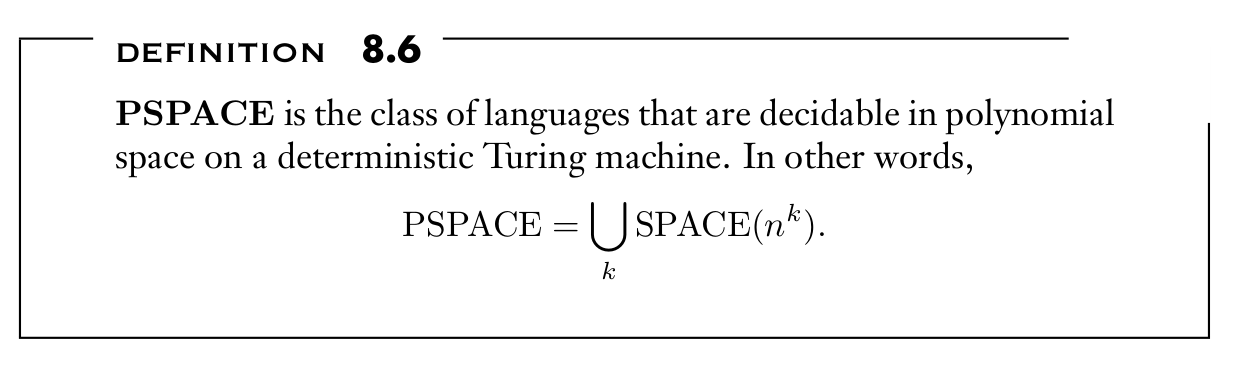class NPSPACE

• equals class PSPACE

relationship with P and NP

• $P ⊆ NP ⊆ PSPACE = NPSPACE ⊆ EXPTIME$

### 8.3 PSPACE-COMPLETENESS

DEF

• using polynomial time reducibility
• the rule is: Whenever we define complete problems for a complexity class, the reduction model must be more limited than the model used for defining the class itself.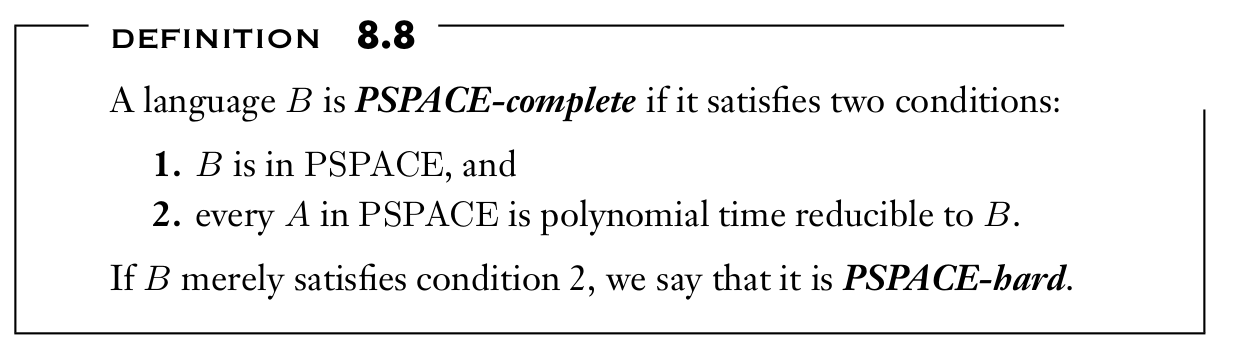THE TQBF PROBLEM

quantified Boolean formulas

• $\phi \ = \ \forall x \ ∃ y \ [ ( x \lor y ) \land ( \bar x \lor \bar y)]$

fully quantified / sentence

• each variable appears within the scope of some quantifier
• always either true or false

$TQBF \ = \ \{ < \phi> | \ \phi \ is \ a \ true \ fully \ quantified \ Boolean \ formula \}$

$TQBF$ is $PSPACE$-complete

Idea

• By DEF: show that every language $A$ in $PSPACE$ is polynomial time reducible to $TQBF$

• Reduction function: takes a string w and produces a quantified Boolean formula φ that simulates the machine for A on input w, while A accept w iff φ is true

• use a technique related to the proof of Savitch’s theorem to construct the formula
• 用A accept w的过程来构造式子 $\phi_{c_{start},{c_{accept}},t}$

Proof

• $T$ deciding $TQBF$ runs in linear space
• if t = 1 construct $\phi_{c_1,c_2,t}$
• $c_1 == c_2$ : same boolean value
• $c_2 \ follows \ c_1$ : Cook-Levin theorem so that $c_1$ correctly yield $c_2$
• if t > 1 construct recursively
• $\phi_{c_1,c_2,t} \ = \ ∃ m_1 [ \phi_{c_1,m_1,\frac{t}{2}} \land \phi_{m_1,c_2,\frac{t}{2}}]$
• reduce size $\phi_{c_1,c_2,t } \ = \ ∃ m_1 \forall ( c_3,c_4) \in \{(c_1,m_1),(m_1,c_2)\} [ \phi_{c_3,c_4,\frac{t}{2}}]$

WINNING STRATEGIES FOR GAMES

formula-game

• $\phi = ∃x_1 ∀x_2 ∃x_3 · · · Qx_k [ ψ ]$
• Player A selects $\forall$ variables and Player E selects $\exists$ variables
• E wins when it’s true and A wins when it’s false

geography game

• go along the directed graph and can’t choose visited nodes
• Player I selects first and Player II second
• loses when unable to continue

winning strategy

• wins when both sides play optimally

$PSPACE$-complete

$FORMULA -GAME \ = \ \{ < \phi>|\ Player \ E \ has \ a \ winning \ strategy \$

​ $in \ the \ formula \ game \ associated \ with \ \phi \}$

$GG \ = \ \{ < G,b>| \ Player \ I \ has \ a \ winning \ strategy \ for \ the \ generalized$

​ $\ geography \ game \ played \ on \ graph \ G \ starting \ at \ node \ b\}$

we can give some evidence for the difficulty of computing optimal play for many board games by generalizing them to an n × n board.

### 8.4 THE CLASSES L AND NL

TM with two tapes

• a read-only input tape, and a read/write work tape
• configuration: without input w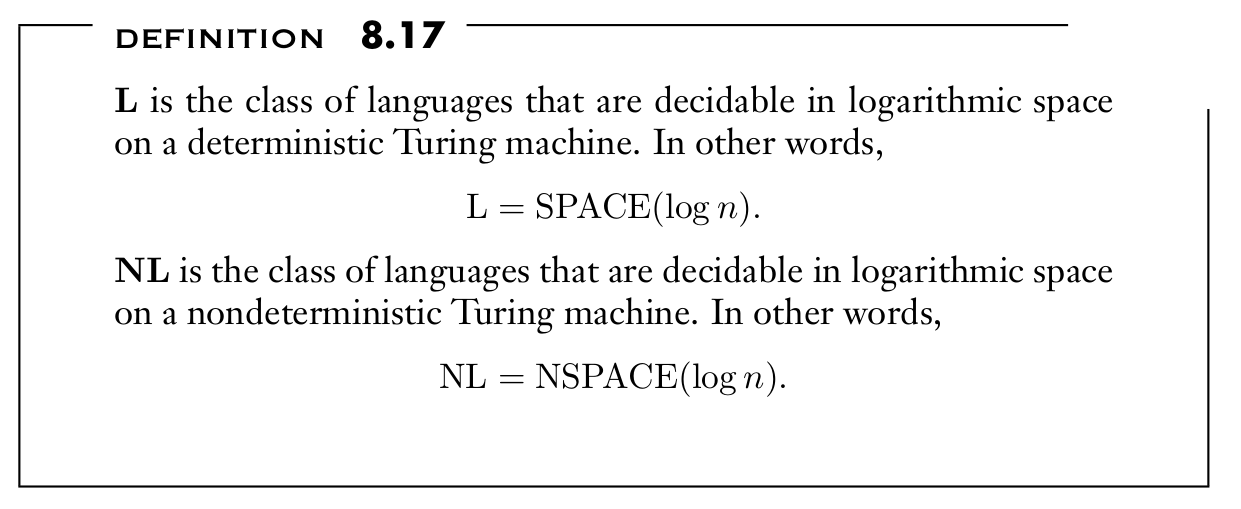Example: $PATH$

### 8.5 NL-COMPLETENESS

LOG SPACE REDUCIBILITY

• the reduction function is computable using $O(logn)$ symbols on work tape
• $A ≤_L B$

DEFINITION OF NL-COMPLETENESS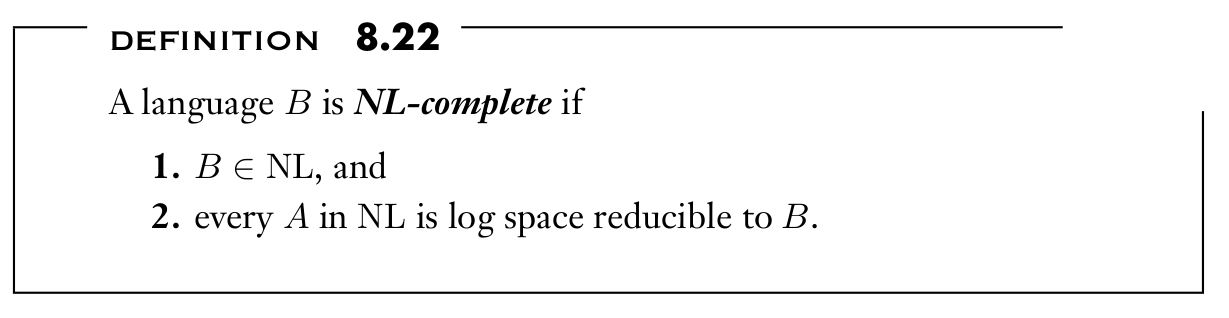THEOREM 8.23

• If $A ≤_L B$ and $B ∈ L$, then $A ∈ L$.

Unknown

• $L = NL$

SEARCHING IN GRAPHS

$PATH = \{ < G, s, t> | \ G \ is \ a \ directed \ graph \ that \ has \ a \ directed \ path \ from \ s \ to \ t\}$

$PATH$ is $NL$-complete

Idea

• construct a graph == the computation of the log space NTM for A

Proof

• nodes: configurations of M on w
• edges: pair (c1 , c2) is an edge of G if c2 is one of the possible next configurations of M starting from c1

COROLLARY 8.26

$NL ⊆ P$

### 8.6 NL EQUALS CONL

$NL = coCL$

Proof

• $\overline { PATH } \in coNL$

•